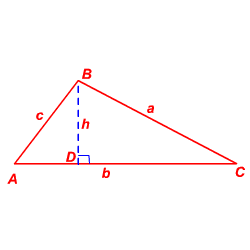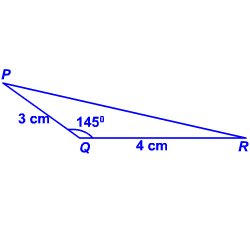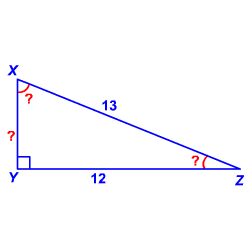# Finding the Area of a Triangle Using Sine

You are familiar with the formula $R=\frac{1}{2}bh$ to find the area of a triangle where $b$ is the length of a base of the triangle and $h$ is the height, or the length of the perpendicular to the base from the opposite vertex.

Suppose $\Delta ABC$ has side lengths $a$ , $b$ , and $c$ . Let $h$ be the length of the perpendicular to the side of length $b$ from the vertex $B$ that meets the side $\stackrel{¯}{AC}$ at $D$ .Then, the area $R$ of the triangle $ABC$ is $R=\frac{1}{2}bh$ .

Now, look at $\Delta ADB$ . It is a right triangle with hypotenuse $\stackrel{¯}{AB}$ that has a length of $c$ units.

Consider the sine of $\angle A$ .

Substituting the value of $h$ in the formula for the area of a triangle, you get

$\begin{array}{l}R=\frac{1}{2}b\left(c\mathrm{sin}\left(A\right)\right)\\ \text{\hspace{0.17em}}\text{\hspace{0.17em}}\text{\hspace{0.17em}}\text{\hspace{0.17em}}\text{\hspace{0.17em}}=\frac{1}{2}bc\mathrm{sin}\left(A\right)\end{array}$

Similarly, you can write formulas for the area in terms of $\mathrm{sin}\left(B\right)$ or $\mathrm{sin}\left(C\right)$ .

$\begin{array}{l}R=\frac{1}{2}ab\mathrm{sin}\left(C\right)\\ R=\frac{1}{2}ac\mathrm{sin}\left(B\right)\end{array}$

Example 1:

Find the area of $\Delta PQR$ .You have the lengths of two sides and the measure of the included angle. So, you can use the formula $R=\frac{1}{2}pr\mathrm{sin}\left(Q\right)$ where $p$ and $r$ are the lengths of the sides opposite to the vertices $P$ and $R$ respectively.

Using the formula the area, $R=\frac{1}{2}\left(3\right)\left(4\right)\mathrm{sin}\left(145°\right)$ .

Simplify.

$\begin{array}{l}R=6\mathrm{sin}\left(145°\right)\\ \text{\hspace{0.17em}}\text{\hspace{0.17em}}\text{\hspace{0.17em}}\text{\hspace{0.17em}}\text{\hspace{0.17em}}\approx 6\left(0.5736\right)\\ \text{\hspace{0.17em}}\text{\hspace{0.17em}}\text{\hspace{0.17em}}\text{\hspace{0.17em}}\text{\hspace{0.17em}}\approx 3.44\end{array}$

Therefore, the area of $\Delta PQR$ is about $3.44$ sq.cm.

Example 2:

The area of the right $\Delta XYZ$ with the right angle at the vertex $Y$ is $39$ sq. units. If $YZ=12$ and $XZ=13$ , solve the triangle.

First, draw a figure with the given measures.Use the Pythagorean Theorem to find the length of the third side of the triangle.

$\begin{array}{l}XY=\sqrt{{\left(XZ\right)}^{2}-{\left(YZ\right)}^{2}}\\ \text{\hspace{0.17em}}\text{\hspace{0.17em}}\text{\hspace{0.17em}}\text{\hspace{0.17em}}\text{\hspace{0.17em}}\text{\hspace{0.17em}}\text{\hspace{0.17em}}\text{\hspace{0.17em}}\text{\hspace{0.17em}}=\sqrt{{13}^{2}-{12}^{2}}\\ \text{\hspace{0.17em}}\text{\hspace{0.17em}}\text{\hspace{0.17em}}\text{\hspace{0.17em}}\text{\hspace{0.17em}}\text{\hspace{0.17em}}\text{\hspace{0.17em}}\text{\hspace{0.17em}}\text{\hspace{0.17em}}=\sqrt{169-144}\\ \text{\hspace{0.17em}}\text{\hspace{0.17em}}\text{\hspace{0.17em}}\text{\hspace{0.17em}}\text{\hspace{0.17em}}\text{\hspace{0.17em}}\text{\hspace{0.17em}}\text{\hspace{0.17em}}\text{\hspace{0.17em}}\text{\hspace{0.17em}}=\sqrt{25}\\ \text{\hspace{0.17em}}\text{\hspace{0.17em}}\text{\hspace{0.17em}}\text{\hspace{0.17em}}\text{\hspace{0.17em}}\text{\hspace{0.17em}}\text{\hspace{0.17em}}\text{\hspace{0.17em}}\text{\hspace{0.17em}}\text{\hspace{0.17em}}\text{\hspace{0.17em}}=5\end{array}$

Now, you have lengths of the three sides and the area of the triangle.

Substitute in the area formula.

$\begin{array}{l}\text{Area}=\frac{1}{2}×\left(YZ\right)×\left(XZ\right)×\mathrm{sin}\left(Z\right)\\ 39=\frac{1}{2}\left(12\right)\left(13\right)\mathrm{sin}\left(Z\right)\end{array}$

Solve for $Z$ .

$\begin{array}{l}\mathrm{sin}\left(Z\right)=\frac{\left(39\right)\left(2\right)}{\left(12\right)\left(13\right)}\\ \text{\hspace{0.17em}}\text{\hspace{0.17em}}\text{\hspace{0.17em}}\text{\hspace{0.17em}}\text{\hspace{0.17em}}\text{\hspace{0.17em}}\text{\hspace{0.17em}}\text{\hspace{0.17em}}\text{\hspace{0.17em}}\text{\hspace{0.17em}}\text{\hspace{0.17em}}\text{\hspace{0.17em}}\text{\hspace{0.17em}}\text{\hspace{0.17em}}\text{\hspace{0.17em}}=0.5\end{array}$

Taking the inverse,

$\begin{array}{l}Z={\mathrm{sin}}^{-1}\left(0.5\right)\\ \text{\hspace{0.17em}}\text{\hspace{0.17em}}\text{\hspace{0.17em}}\text{\hspace{0.17em}}\text{\hspace{0.17em}}=30°\end{array}$

That is, $m\angle Z=30°$ .

Given that the angle at the vertex $Y$ is a right angle. Therefore, $m\angle Y=90°$ .

Using the Triangle Angle Sum Theorem , the measure of the third angle is,

$\begin{array}{l}m\angle X=180-\left(m\angle Y+m\angle Z\right)\\ \text{\hspace{0.17em}}\text{\hspace{0.17em}}\text{\hspace{0.17em}}\text{\hspace{0.17em}}\text{\hspace{0.17em}}\text{\hspace{0.17em}}\text{\hspace{0.17em}}\text{\hspace{0.17em}}\text{\hspace{0.17em}}\text{\hspace{0.17em}}\text{\hspace{0.17em}}\text{\hspace{0.17em}}\text{\hspace{0.17em}}=180-\left(90+30\right)\\ \text{\hspace{0.17em}}\text{\hspace{0.17em}}\text{\hspace{0.17em}}\text{\hspace{0.17em}}\text{\hspace{0.17em}}\text{\hspace{0.17em}}\text{\hspace{0.17em}}\text{\hspace{0.17em}}\text{\hspace{0.17em}}\text{\hspace{0.17em}}\text{\hspace{0.17em}}\text{\hspace{0.17em}}\text{\hspace{0.17em}}=60\end{array}$

Therefore, the measure of $\angle X$ is $60°$ .